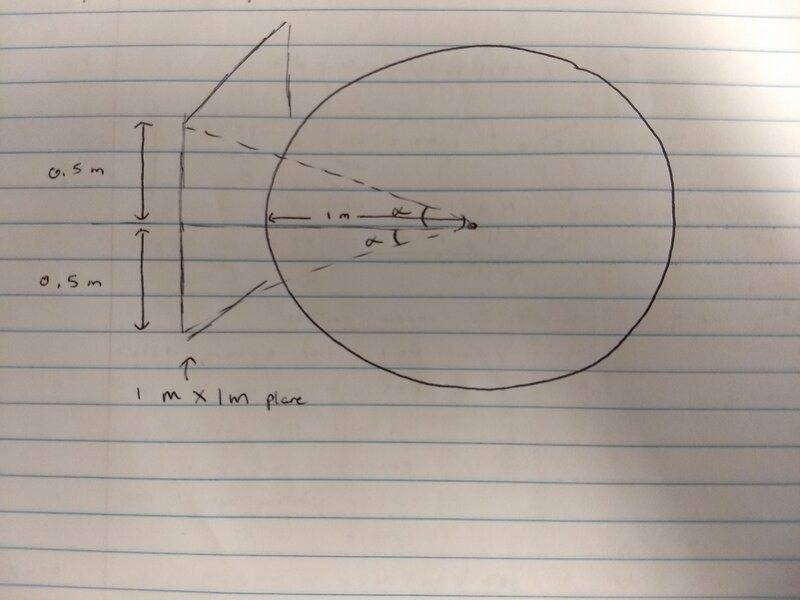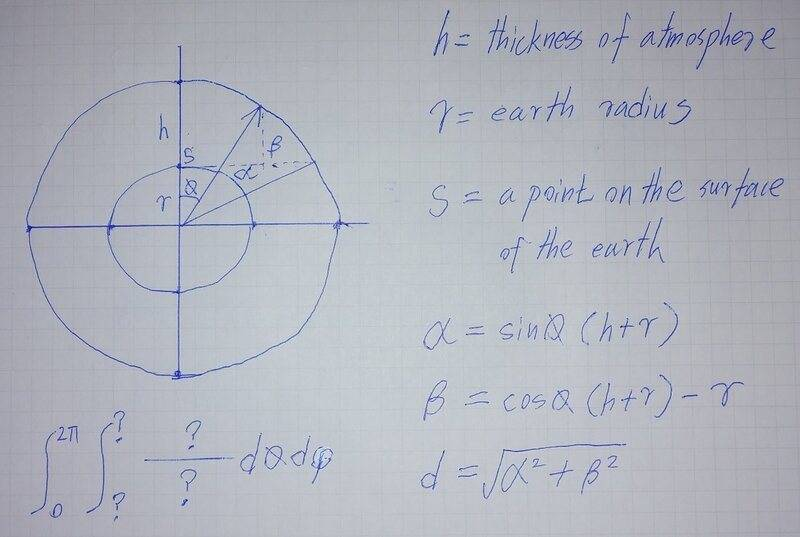# Solid angle in an optics problem (artificial irradiance of the Earth)

baseballfan_ny
Homework Statement:
How many commercially available light bulbs of 100W each placed at 1m away from the
atmosphere’s edge would generate an irradiance of 1370 W/m^2 on an area of 1m2?
Relevant Equations:
Solid angle = spherical volume integral/rho^2
The actual problem can be found as #2 on this link: https://ocw.mit.edu/courses/mechani...s-spring-2009/assignments/MIT2_71S09_ups1.pdf

I rewrote the problem above with the solar irradiance data that they give.My interpretation is of a square 1 m x 1 m plane sitting behind an imaginary sphere. So the angle ##\alpha = \arctan(0.5)##. The angle swept by phi in the vertical direction should go from ##-\alpha## to ##+\alpha## and the angle swept by theta radially in the xy plane should also be the same given the square shape.

The solid angle of a sphere is ##4\pi##, so the ratio of the solid angles I need is $$\frac {\Omega} {4\pi} = \frac {1370} {100 N}$$

where N is the number of lightbulbs.

Now for calculating the solid angle ##\Omega## I am getting tripped up.
$$d\Omega = \sin(\phi)d\phi d\theta$$
$$\Omega = \int_{\theta = -\alpha}^{\alpha} \int_{\phi = -\alpha}^{\alpha} \sin(\phi) \, d\phi \, d\theta$$
$$\Omega = 2\alpha * (-\cos(\alpha) - - \cos(-\alpha))$$
$$\Omega = 2\alpha * (-\cos(\alpha) + \cos(\alpha))$$
$$\Omega = 0 ?!?$$

I know that can't be right
Ω

Homework Helper
Gold Member
2022 Award
You are making the problem unnecessarily complicated. At the distance of the bulbs from the surface, the illuminated area of one square metre might as well be a cap of your imaginary sphere, not a flat sheet. In other words, you just want the intensity of power falling on your sphere.

Wrt to your attempt, your first equation is dimensionally wrong. On the left you have the ratio of two solid angles, on the right a power intensity ##(W/m^2)## divided by power (W).

Edit: I may have misinterpreted the question.

Last edited:
baseballfan_ny
Wrt to your attempt, your first equation is dimensionally wrong. On the left you have the ratio of two solid angles, on the right a power intensity (W/m2) divided by power (W).
Well, ok. I guess since we're dealing with an area of I square meter, the power over that area is ## 1370 [W m^{-2}] * 1 [m^2] = 1370 [W] ##, so I could think of it as a ratio of two powers (or two intensities since the intensity of ##100N [W]## over 1 square meter is also ##100N [W m^{-2}]##). So I don't think I technically have to change the ratio, just have to keep all that in mind?

You are making the problem unnecessarily complicated. At the distance of the bulbs from the surface, the illuminated area of one square metre might as well be a cap of your imaginary sphere, not a flat sheet. In other words, you just want the intensity of power falling on your sphere.
I think I am going for the intensity on the sphere ... that's why I did the integral. I only made the plane to try to get the bounds of integration for the solid angle ... I'm assuming the bounds are messed up?

baseballfan_ny
Would it be valid to just ignore the integral and everything and use ## \Omega = \frac {Area} {r^2} ##, so ##\Omega = 1 ## because we have a 1 m^2 area over a radius of 1 m?

And then $$\frac {1} {4\pi} = \frac {1370} {100N}$$
$$N = 1370 * 4\pi /100$$
$$N \approx 172$$

Homework Helper
Gold Member
2022 Award
Would it be valid to just ignore the integral and everything and use ## \Omega = \frac {Area} {r^2} ##, so ##\Omega = 1 ## because we have a 1 m^2 area over a radius of 1 m?
I may have misunderstood the question. You are assuming all the light bulbs are concentrated at a single point, which may be right.
If so, no, your original approach was right, but your integral was wrong. The integrand assumes theta and phi represent spherical polar coordinates, but if you project a portion of of a sphere's surface between a range of latitudes and a range of longitudes out onto a plane you will not get a straight-sided figure.
Instead, let's try some geometry. You have a pyramid on a square base. Can you figure out the angles between adjacent triangular faces?

Edit: you probably have not been taught enough geometry to solve it this way so I'll give a bit more help.

Consider a right pyramid on a square base. The sides of the base are 2x and the height of the pyramid y.
Label the top of the pyramid O and the corners of the base ABCD.
Drop a perpendicular from A to meet OB at P and one from O to meet AB at Q.
We need to find angle ##\theta##=APC. You could try that bit yourself, but here are more hints...
We have AB.OQ=AP.OB, i.e. ##2x\sqrt{y^2+x^2}=AP.\sqrt{y^2+2x^2}##, or ##4x^2(y^2+x^2)=AP^2(y^2+2x^2)##.
AC is easy to find, and hence theta using the cosine rule on triangle APC.

Why is theta interesting? The sides of the pyramid project out onto a sphere, cutting it in four arcs. The angle between two adjacent arcs is theta (think about it). We can now apply a theorem relating the angles of a figure on the surface of a sphere to the solid angle it subtends at the sphere's centre.
For a triangle, the formula is ##\alpha+\beta+\gamma=\pi+\Omega##. Extend that to the "square".

Last edited:
baseballfan_ny
We have AB.OQ=AP.OB, i.e. 2xy2+x2=AP.y2+2x2, or 4x2(y2+x2)=AP2(y2+2x2).
How are you getting this? I'm not sure I'm familiar with what theorem this is.

I tried finding ##\theta## = APC before reading those hints and ended up taking a longer approach and getting a different value for AP. I got AP = ## \frac {\sqrt{3(y^2 + x^2)}} {2} ## with an end result of ##\theta = \arccos{1 - \frac {16x^2} {5(x^2 + y^2)}}##.

My process for finding AP was to use the right triangle formed by OAP:

$$AP = \sqrt{OA^2 - OP^2}$$

And OP = OA/2

$$AP = \sqrt{\frac {3OA^2} {4}} = \frac {OA} {2} * \sqrt{3}$$

OA can be found using Pythag on the height of triangle (y) and half the diagonal of the base (##\sqrt{2}x##), which gives OA = ##\sqrt{y^2 + 2x^2}##, so then ...

$$AP = \frac {\sqrt{3(y^2 + x^2)}} {2}$$

Homework Helper
Gold Member
2022 Award
How are you getting this?
OB is one side of triangle OAB, and AP is normal to it. So the area of the triangle is OB.AP/2. Likewise, it is OQ.AB/2.
OP = OA/2
You must have the wrong diagram.

Homework Helper
Gold Member
"How many commercially available light bulbs placed at 1 m away from the atmosphere's edge would generate the same amount of irradiance on an area of 1 m2?"

The 1 meter is from the atmosphere's edge not from the surface of the Earth. A number of lightbulbs are placed at the edge of the atmosphere and presumably are to be considered a point source. The problem does not specify the bulb wattage, but this question is under the title "order of magnitude calculations" so 100 W is fine. One needs to look up the distance ##r## from the atmosphere's edge to the surface of the Earth, figure out the solid angle subtended by an area of 1 square meter and then do the necessary calculation.

Homework Helper
Gold Member
2022 Award
"How many commercially available light bulbs placed at 1 m away from the atmosphere's edge would generate the same amount of irradiance on an area of 1 m2?"
Yes, but it does not say where the area of 1sq m is. The reference to "the same amount" presumably relates to "incident outside the earth’s atmosphere", suggesting the benchmark is the irradiation incident on the atmosphere.
Nor does it define the height of the atmosphere - is there a recognised value for that?
So to me it is highly ambiguous.

OTOH, I do doubt the solver is expected to go through this solid angle calculation.

Homework Helper
Gold Member
Nor does it define the height of the atmosphere - is there a recognised value for that?
So to me it is highly ambiguous.
Yes, I read more to the question than there is. This is an order of magnitude calculation and the neighboring questions are rather elementary. The edge of the atmosphere is mentioned only for the ~1300 W/m2 number that can be read from the plot. It looks like a simple division problem.

Homework Helper
Gold Member
2022 Award
It looks like a simple division problem.
But if we take the r=1m interpretation, what division, exactly? There being no mention of a mirror, at least half the power would be radiated out to space. Quite a lot would miss the 1sqm target.
Besides, the geometric method for finding the solid angle is fun, and quite instructive, so at least there is some value in going that path.

alan123hk
I guess it may be similar to the following situation.Homework Helper
Gold Member
But if we take the r=1m interpretation, what division, exactly? There being no mention of a mirror, at least half the power would be radiated out to space. Quite a lot would miss the 1sqm target.
Besides, the geometric method for finding the solid angle is fun, and quite instructive, so at least there is some value in going that path.
A factor of half is meaningless in an order of magnitude calculation. Besides, as you pointed out there are many unspecified factors, e.g. bulb wattage, bulb efficiency, collimation or no collimation, etc. I think one has to rise above such details and try to divine the intent of the question's author before answering it. My guess is that, as an order of magnitude calculation, it is trying to establish a connection between an unfamiliar number and a familiar number. In this case, the unfamiliar number is the 1300 W/m2 and the familiar number is the output of a commercially available lightbulb, the kind that is used to illuminate rooms. The question asks how many of these would be required to produce an irradiance equivalent to the Sun's on the Earth's atmosphere. The order of magnitude answer would be 10 100-W bulbs in a square array 1 m on the side. They could be 16 (to form a perfect square) but not 1 or 100. That's the sense I make of this question.

Homework Helper
Gold Member
2022 Award
A factor of half is meaningless in an order of magnitude calculation.
But a factor of 1/15 (exactly, ##\frac{4\arccos(-\frac 15)-2\pi}{4\pi}##) is not.

alan123hk
I really don't understand the meaning of this question. The question does not define the actual value of the atmosphere height, and what does it mean to be 1m away from the atmosphere’s edge ?

I believe the following two situations may be easier to understand.

How many bulbs of 100W one meter away from each other (one light bulb per square meter on average) are placed on the edge of the atmosphere will produce the same amount of irradiance (1370 watts/square meter) on the earth’s surface?

How many light bulbs of 100W evenly placed on the edge of the atmosphere will produce the same amount of irradiance (1370 watts/square meter) on the surface of the earth?

https://earthobservatory.nasa.gov/features/EnergyBalance/page2.php
https://www.e-education.psu.edu/earth103/node/1004

Last edited:
•